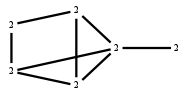The Markov Bases Database

G140g_bin

The binary graphical model of G140.

It is a hierarchical model of 6 variables. The dimension of the model is 16.The cardinality of the statespace is 64.

Properties of the Markov basis

 Markov degree 4 1736

 degree # of gen. 2 4 352 1384

The model has the following properties:

• All variables are binary.
• It is a graphical model.
• The semigroup is normal.

The following properties of the model are unknown:

• The minimal Markov basis may be unique.FILES

 Markov basis: sufficient statistics matrix: G140g_bin.mar (327.20 kb) G140g_bin.mat (3.53 kb) G140g_bin.mod (51 b) G140g_bin.tar.gz (18.47 kb)

Wrong or missing information? Write us an email.

page last update on November 15 2020. ->Contact